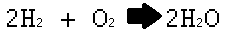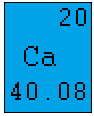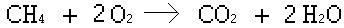# Using Moles in Chemical Equations

Instructor: Laura Foist

Laura has a Masters of Science in Food Science and Human Nutrition and has taught college Science.

In this lesson we will explore chemical equations and what we can learn about them using moles. We will learn what a mole is and how moles are used to determine the mass needed for a reaction to occur.

## What Are Moles?

In chemistry we frequently use the word 'moles' to refer to the amount of chemicals used. This mole is not the little animal that digs around in your yard, instead it refers to a set quantity of atoms.

Think of it like the word 'dozen'. When you say two dozen eggs, you mean two sets of 12 eggs. Moles represent a much bigger number though, 6.023 times ten to the 23rd, which is called 'Avogadro's number'. So instead of saying, 'I have a dozen atoms' we can say 'I have an Avogadro's number of atoms' (in chemistry we don't typically say it like that, but it's a good way to think about what a mole means).

Remember, we don't just react a single molecule with another single molecule, or even a dozen (that would be such a small reaction that we wouldn't be able to see it). Instead, this is happening on very large scale, which is why we often use such a large number such as Avogadro's number to talk about these reactions.

The chemical equation will tell us the number of moles that are used, which in turn can tell us the mass and volume.

## Moles in Chemical Equations

Now let's think about the dozen atoms again in regard to the water reaction and moles. Here is the chemical equation for making water:We see that 2 molecules of hydrogen reacts with 1 molecule of oxygen to form 2 molecules of water. Now typically we aren't only making 1 molecule (remember, that would be on such a small quantity that we wouldn't be able to see it let alone measure it out).

So let's double it. This would mean that we are using 4 molecules of hydrogen to react with 2 molecules of oxygen to form 4 molecules of water. How about a dozen times of each? 2 dozen hydrogen molecules and 1 dozen oxygen molecules makes 2 dozen water molecules. So really, it is 24 hydrogen molecules reacting with 12 oxygen molecules to make 24 water molecules, but instead of saying 24, 12, and 24 we can look at it as multiples of a dozen.

The same terminology is used for moles. We can say we use 2 moles of hydrogen and 1 mole of oxygen to make 2 moles of water. Instead of saying we are using 6.023 times ten to the 23rd oxygen molecules we can simply say that we are using 1 mole of oxygen. This is the way that chemists typically refer to chemical equations.

## Moles and the Periodic Table

Sometimes a periodic table will simply have the names or abbreviations for each element in the blocks. But sometimes we will see numbers in these blocks. Let's look at calcium.We know that the Ca is simply the abbreviation for calcium. But what are those numbers? The '20' in the top right corner simply refers to the fact that calcium is element number 20. The 40.08 on the bottom is the important number right now. This is the atomic weight of calcium. The atomic weight of each element is the weight (in grams) of 1 mole of that particular element.

## Using Moles to Determine Mass

The nice thing about using moles to talk about chemical equations is that we know the weight of each element in a mole. We can determine the weight of a molecule simply by adding all of the weights for each atom.

Now let's look at a chemical equation:To unlock this lesson you must be a Study.com Member.

### Register to view this lesson

Are you a student or a teacher?

### Unlock Your Education

#### See for yourself why 30 million people use Study.com

##### Become a Study.com member and start learning now.
Back
What teachers are saying about Study.com

### Earning College Credit

Did you know… We have over 160 college courses that prepare you to earn credit by exam that is accepted by over 1,500 colleges and universities. You can test out of the first two years of college and save thousands off your degree. Anyone can earn credit-by-exam regardless of age or education level.